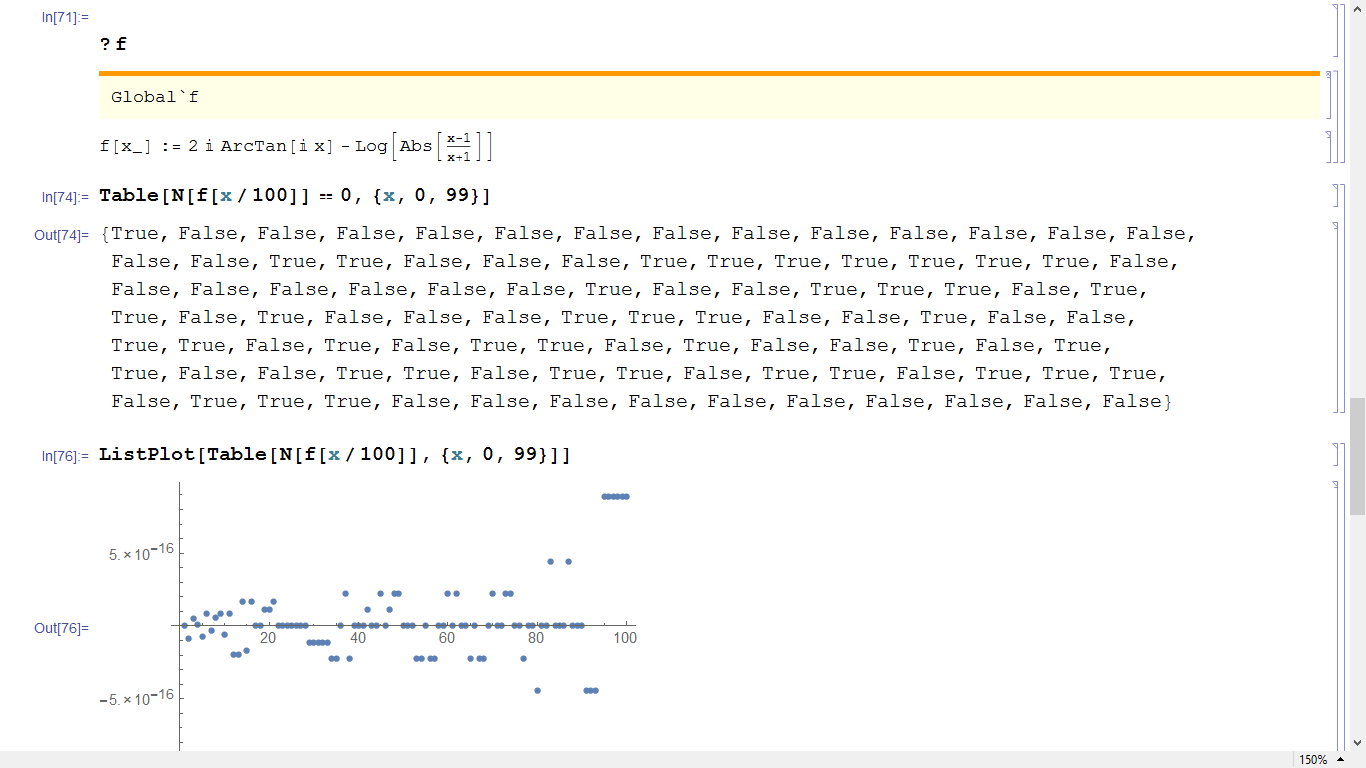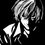# An Elegant Solution to a Complex ProblemBefore trying to solve the problem I present here in this note, please make sure that you have read the complete note clearly.

Problem. Prove $2i\tan^{-1} \left(\frac{ia}{b}\right) = \log \left|\frac{a-b}{a+b}\right|$ where $$i = \sqrt{-1}$$ and $$a,b \in \mathbb{R}$$ such that $$a \neq \pm b$$

Proof. Ofcoure, you can prove it in a several ways, like simplifying using complex analysis or using series expansion or maybe some other method(s).

But I'll share another elegant solution to the above problem.

Consider the following integral

$\int_0^x \frac{1}{x^2-a^2} \mathrm{d}x = \frac{1}{2a}\int_0^x \frac{1}{x-a} - \frac{1}{x+a} \mathrm{d}x = \frac{1}{2a} \log \left|\frac{x-a}{x+a}\right|$

We can also evaluate the above integral as $\int_0^x \frac{1}{x^2-a^2} \mathrm{d}x = \int_0^x \frac{1}{x^2+(ia)^2} \mathrm{d}x = \frac{i}{a}\tan^{-1} \left(\frac{ix}{a}\right)$

Thus, $\frac{i}{a} \tan^{-1} \left(\frac{ix}{a}\right) = \frac{1}{2a} \log \left|\frac{x-a}{x+a}\right|$ $\Rightarrow 2i \tan^{-1} \left(\frac{ix}{a}\right) = \log \left|\frac{x-a}{x+a}\right| \qquad \qquad \qquad \square$

Now, here I have a problem. When I tried to verify the above result on Mathematica, it gives me the negative result. Here's a screenshot of my code (in particular, I did it for $a=1$ and for $|x| <1$). I expected Mathematica to return true for all values.

Can somebody help me, where am I going wrong. Is there a flaw in the result that I have proven , or is my Mathematica code wrong?Note by Kishlaya Jaiswal
6 years, 4 months ago

This discussion board is a place to discuss our Daily Challenges and the math and science related to those challenges. Explanations are more than just a solution — they should explain the steps and thinking strategies that you used to obtain the solution. Comments should further the discussion of math and science.

When posting on Brilliant:

• Use the emojis to react to an explanation, whether you're congratulating a job well done , or just really confused .
• Ask specific questions about the challenge or the steps in somebody's explanation. Well-posed questions can add a lot to the discussion, but posting "I don't understand!" doesn't help anyone.
• Try to contribute something new to the discussion, whether it is an extension, generalization or other idea related to the challenge.

MarkdownAppears as
*italics* or _italics_ italics
**bold** or __bold__ bold
- bulleted- list
• bulleted
• list
1. numbered2. list
1. numbered
2. list
Note: you must add a full line of space before and after lists for them to show up correctly
paragraph 1paragraph 2

paragraph 1

paragraph 2

[example link](https://brilliant.org)example link
> This is a quote
This is a quote
    # I indented these lines
# 4 spaces, and now they show
# up as a code block.

print "hello world"
# I indented these lines
# 4 spaces, and now they show
# up as a code block.

print "hello world"
MathAppears as
Remember to wrap math in $$ ... $$ or $ ... $ to ensure proper formatting.
2 \times 3 $2 \times 3$
2^{34} $2^{34}$
a_{i-1} $a_{i-1}$
\frac{2}{3} $\frac{2}{3}$
\sqrt{2} $\sqrt{2}$
\sum_{i=1}^3 $\sum_{i=1}^3$
\sin \theta $\sin \theta$
\boxed{123} $\boxed{123}$

Sort by:

i solved the problem in the exact manner ! it was in Arihant level 2 complex problems right?

I too solved it through integration and later i realised that using demoures theorem (means $e^{ix}$ was much easier ,

- 6 years, 4 months ago

I derived this integral in some boring class and thought it was something great. Then I found out that using $e^{i\theta}$, this thing is very elementary.

- 6 years, 4 months ago

@Mvs Saketh @Raghav Vaidyanathan But did you notice the last line of the note.

Mathematica doesn't gives this result, can you tell me the flaw in my code?

- 6 years, 4 months ago

I'm sorry, I do not know mathematica. But I do know that wolfram if not error free. Your result seems correct to me, but I am not able to see why you are getting false values.

- 6 years, 4 months ago

See my comment for an explanation.

- 6 years, 4 months ago

- 6 years, 4 months ago

The fallacy is in the definite integrals.

In a similar way, one could prove that sin^2(x) = -cos^2(x)

$\int^x_0{\sin x \cos x \, dx} = \int^x_0{(\sin x) d(\sin x) } = \frac{1}{2} \sin^2 x$

$\int^x_0{\sin x \cos x \, dx} = \int^x_0{-(\cos x) d(\cos x) } = -\frac{1}{2} \cos^2 x$

$\implies \sin^2 x = - \cos^2 x$

- 6 years, 4 months ago

Actually your last integral is incorrect. Let me explain

$\int_0^x \sin x \cos x dx = \int_0^1 -(\cos x)d(\cos x) = -\frac{1}{2}\left(\cos^2 x\right|_0^x = -\frac{1}{2}\left(\cos^2 x - 1\right) = \frac{1}{2}(1-\cos^2 x) = \frac{1}{2} \sin^2 x$

- 6 years, 4 months ago

Sorry to confuse you, I don't think the problem is with the integral.

The problem is with the N function. Because it forces the software to convert both sides to numeric expressions, it is unlikely that they'll be the same.

I am not exactly sure why it happens but something like this might happen: Mathematica's calculation of one side may converge faster than the other. For example, if both sides are $\sqrt{2}$, one might converge to 1.41 and another to 1.414 after a certain number of iterations.

In computation, many a times a 0 and a small 'weed' error are often indistinguishable.

To make sure what I am talking about is not crap, run the following:

 1 2 3 4 f[x_] := 2 I ArcTan[(I x)/1]; g[x_] := Log[Abs[(x - 1)/(x + 1)]]; Table[f[x/100] - g[x/100] // N, {x, 0, 99}] 

See how small the values are?

Okay, let us resort to symbolic computation!

 1 2 3 f[x_] := 2 I ArcTan[(I x)/1]; g[x_] := Log[Abs[(x - 1)/(x + 1)]]; Table[f[x/100] - g[x/100] == 0 // FullSimplify, {x, 0, 99}] 

Run the code! Does it satisfy you?

- 6 years, 4 months ago

Oh,yeah, now I see my flaw. Thanks

And also,

 1 Solve[2*I*ArcTan[(*x)/1] - Log[Abs[(x - 1)/(x + 1)]] == 0,x] 

gives me all real solutions.

A great thanks to you for pointing it out. $\ddot \smile$

- 6 years, 4 months ago

You are Welcome. :)

- 6 years, 4 months ago

Actually, there shouldn't be a fallacy because I found that both the sides of expression have same series expansion.

Also, if still there is fallacy, then can you please pin-point the wrong step.

And please do share the fallacy which proves that $\sin^2x = \cos^2x$

- 6 years, 4 months ago

I'm not sure of the exact fallacy but check the last comment again :)

- 6 years, 4 months ago

With regards to your fallacy, that's not what you want. What you intended is

$\int \sin x \cos x \, dx = \int \sin x \, d \sin x = \frac{1}{2} \sin ^ 2 x$
$\int \sin x \cos x \, dx = \int - \cos x \, d \cos x = - \frac{1}{2} \cos ^ 2 x$

Hence, this would lead someone to claim that $\sin^2 x = - \cos ^2 x$.

This is a common example used to represent this mistake, which is that $\int f \, dx = F(x) + C$, as opposed to $\int f\, dx = F(x)$. This appears numerous times, even on Brilliant. E.g. we've had to delete a lot of questions that say " Evaluate $\int f\, dx$ at $x = 0$", because they didn't make sense due to the $+ C$ which was forgotten.

Do you see how the mistake comes into play here?

Staff - 6 years, 4 months ago

Yep, the constant is -1/2 ;)

- 6 years, 4 months ago

@bobbym none I'd like you to throw some light on this

- 6 years, 4 months ago